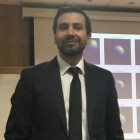﻿﻿ CI²MA - Publications | Graduate Thesis

# Graduate Thesis of Daniel InzunzaProgramPhD in Applied Sciences with mention in Mathematical Engineering, Universidad de Concepción
Enrollment Year2013
Senior Year2019
Thesis TitleImplicit-Explicit Methods for Non-Linear and Non-Local Convection-Diffusion-Reaction Problems

#### Thesis Summary:

In this thesis, high-order numerical methods are used to approximate the solution of nonlinear and nonlocal equations with gradient-type flow structure. Specifically, numerical schemes are proposed for aggregation models and for convection-diffusion problems. The thesis has the following objectives. The first objective of this thesis is to propose a high-order scheme for a non-linear and non-local equation with gradient flow, analyzing its properties and applications for both the one-dimensional and the multidimensional case. The second objective of this thesis is to show that the implicit-explicit Runge-Kutta (IMEXRK) schemes allow to obtain an efficient numerical solution of both the generated error and also the CPU time for convection-diffusion problems with nonlocal and nolinear terms. These schemes consist in handling the convective part by treating Runge-Kutta schemes, and the diffusive part by implicit schemes. For the latter, by discretizing the resulting implicit scheme, a system of nonlinear equations is obtained, which solved by the Newton-Raphson method with descent algorithm. The obtained scheme allows a less restrictive CFL condition compared with an explicit scheme. The third objective of this thesis is to show an application of high-order schemes to population dynamics and pedestrian movement models. It turns out that for coarse discretizations of the computational mesh the numerical solutions obtained are more sharply resolved than those obtained with first-order schemes.

Thesis Director(s) Raimund Bürger, Pep Mulet, Luis M. Villada
Thesis Project Approval Date2016, November 22
Thesis Defense Date2019, December 16
Professional Monitoring
PDF ThesisDownload Thesis PDF#### ISI Publications from the Thesis

Raimund BüRGER, Daniel INZUNZA, Pep MULET, Luis M. VILLADA: Implicit-explicit methods for a class of nonlinear nonlocal gradient flow equations modelling collective behaviour. Applied Numerical Mathematics, vol. 144, pp. 234-252, (2019).

Raimund BüRGER, Daniel INZUNZA, Pep MULET, Luis M. VILLADA: Implicit-explicit schemes for nonlinear nonlocal equations with a gradient flow structure in one space dimension. Numerical Methods for Partial Differential Equations, vol. 35, 3, pp. 1008-1034, (2019).

<< Back to list of Graduate Thesis.

 CI²MA, CENTER FOR RESEARCH IN MATHEMATICAL ENGINEERING, UNIVERSIDAD DE CONCEPCIÓN - MAILBOX 160-C, CONCEPCIÓN, CHILE, PHONE: +56-41-2661324